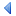UpTOC Index << 3 Using Euphoria < 4.2 Declarations Up: 4 Language Reference 4.4 Branching Statements > 5 Formal Syntax >>

## 4.3 Assignment statement

An assignment statement assigns the value of an expression to a simple variable, or to a subscript or slice of a variable. e.g.

```x = a + b
y[i] = y[i] + 1
y[i..j] = {1, 2, 3}
```

The previous value of the variable, or element(s) of the subscripted or sliced variable are discarded. For example, suppose x was a 1000-element sequence that we had initialized with:

```object x

x = repeat(0, 1000)  -- a sequence of 1000 zeros
```

and then later we assigned an atom to x with:

```x = 7
```

This is perfectly legal since x is declared as an object. The previous value of x, namely the 1000-element sequence, would simply disappear. Actually, the space consumed by the 1000-element sequence will be automatically recycled due to Euphoria's dynamic storage allocation.

Note that the equals symbol '=' is used for both assignment and for equality testing. There is never any confusion because an assignment in Euphoria is a statement only, it can't be used as an expression (as in C).

### 4.3.1 Assignment with Operator

Euphoria also provides some additional forms of the assignment statement.

To save typing, and to make your code a bit neater, you can combine assignment with one of the operators:

```+ - / * &
```

```mylongvarname = mylongvarname + 1
```

You can say:

```mylongvarname += 1
```

```galaxy[q_row][q_col][q_size] = galaxy[q_row][q_col][q_size] * 10
```

You can say:

```galaxy[q_row][q_col][q_size] *= 10
```

```accounts[start..finish] = accounts[start..finish] / 10
```

You can say:

```accounts[start..finish] /= 10
```

In general, whenever you have an assignment of the form:

```left-hand-side = left-hand-side op expression
```

You can say:

```left-hand-side op= expression
```

where op is one of:

```+ - * / &
```

When the left-hand-side contains multiple subscripts/slices, the op= form will usually execute faster than the longer form. When you get used to it, you may find the op= form to be slightly more readable than the long form, since you don't have to visually compare the left-hand-side against the copy of itself on the right side.

You cannot use assignment with operators while declaring a variable, because that variable is not initialized when you perform the assignment.UpTOC Index << 3 Using Euphoria < 4.2 Declarations Up: 4 Language Reference 4.4 Branching Statements > 5 Formal Syntax >>